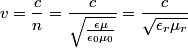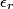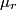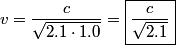## Solution to 1986 Problem 3

 The speed of electromagnetic radiation in matter is\begin{align*}v = \frac{c}{n} = \frac{c}\sqrt{\frac{\epsilon \mu}{\epsilon_0 \mu_0}}} = \frac{c}{\sqrt{\epsilo..$\epsilon_r$, the relative permittivity (also known as the dielectric constant), is 2.1 and$\mu_r$, the relative permeability, is 1.0, so\begin{align*}v = \frac{c}{\sqrt{2.1 \cdot 1.0}} = \boxed{\frac{c}{\sqrt{2.1}}}\end{align*}Hence, answer (D) is correct.